Mechanics

# Mechanical energy

Mechanical energy is a scalar quantity measured in joules. It corresponds to the result of the sum of the kinetic energy and the potential energy of a system. In conservative systems, that is, those that do not present friction or drag forces , the total mechanical energy is conserved.

## Mechanical energy formula

As mentioned earlier, mechanical energy is equal to the sum of kinetic energy and potential energy , check: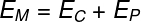M – mechanical energy

C – kinetic energy

P – potential energy

Kinetic energy is related to motion, while potential energy is related to the position in which a body is . There are several types of potential energy, however, in the field of mechanics , we usually do calculations with gravitational potential energy and elastic potential energy.

Next, we will detail the formulas for kinetic energy and elastic and gravitational potential energies.

## kinetic energy formula

The formula for kinetic energy depends on the mass of the body , in kg, and the velocity of the body , in m/s, but it can also be written in terms of momentum , as shown below: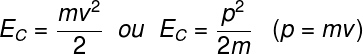C – kinetic energy

m – mass

v – speed

p – amount of movement

Gravitational potential energy formula

Gravitational potential energy is that contained in bodies that have some height in relation to the Earth, due to gravity . The formula for gravitational potential energy is shown below: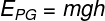The mechanical energy in the conservative system is equal at all points.

PG – gravitational potential energy

m – mass

g – acceleration due to gravity

h – height

## Elastic potential energy formula

Elastic potential energy arises when we deform some elastic body , like a rubber band, for example . This form of energy depends on the deformation , that is, on the increase suffered by the body, but also on the spring constant k .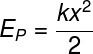The mechanical energy in the conservative system is equal at all points.

k – spring constant (N/m)

x – deformation (m)

## Conservation of mechanical energy

When there are no dissipative forces , the magnitude of the mechanical energy remains constant , in other words, there are no losses of mechanical energy , since the frictional force, for example, converts mechanical energy into thermal energy .

Look at the following figure, if the system represented by it is conservative, then the mechanical energy must be equal at points A, B, taken arbitrarily:

In this way, we can say that two different positions of a conservative system, A and B, for example, present exactly the same mechanical energy, therefore:

## Solved mechanical energy exercises

In all exercises, consider the acceleration of gravity to be g = 10 m/s².

Question (Udesc) An object of mass 500g is dropped from a height of 5m above the ground. Mark the alternative that represents the speed of the object, immediately, before touching the ground, neglecting air resistance.

a) 10 m/s

b) 7.0 m/s

c) 5.0 m/s

d) 15 m/s

e) 2.5 m/s

Template : Letter A

Resolution :

Let’s use the conservation of mechanical energy so that, through kinetic energy and gravitational potential energy, we can find out with what speed the object reaches the ground, check: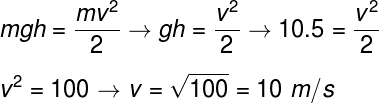The above calculation can be done taking into account that, at the point where it was released, the object had only gravitational potential energy and, immediately before touching the ground, only kinetic energy. Based on the result obtained, the correct alternative is letter A.

Question 2) (Ifsp) A pole vaulter, during his race to overcome the obstacle in front of him, transforms his ____________ energy into ____________ energy due to the height gain and consequently to the _____________ of his speed.

The gaps in the text above are correctly and respectively filled in by:

a) potential – kinetics – increase.

b) thermal – potential – decrease.

c) kinetic – potential – decrease.

d) kinetic – thermal – increase.

e) thermal – kinetic – increase.

Template : Letter C

Resolution :

Check the sentence correctly filled in:

A pole vaulter, during his race to cross the obstacle in front of him, transforms his kinetic energy into potential energy due to the increase in height and consequently to the decrease in his speed.

Question 3) (Ifba) The Beach Park, located in Fortaleza-CE, is the largest water park in Latin America located by the sea. One of its main attractions is a water slide called “Insano”. Going down this water slide, a person reaches its lowest point with a speed of 28 m/s.

Considering the acceleration of gravity with magnitude g = 10 m/s² and ignoring friction, it is estimated that the height of the water slide, in meters, is:

a) 28

b) 274.4

c) 40

d) 2.86

e) 32

Template : Letter C

Resolution :

The exercise requires that we apply the principle of conservation of mechanical energy, for that, the mechanical energy at the top of the waterslide must be equal to the mechanical energy at its base, in the case of energies of a purely potential and kinetic nature, respectively, thus, just do the following calculation: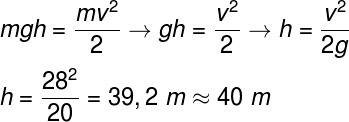The calculations indicate that the height of the water slide is approximately 40 m, so the correct alternative is the letter C.